Theory and Modern Applications

# A unified approach to nonlocal impulsive differential equations with the measure of noncompactness

## Abstract

This paper is concerned with the existence of mild solutions to impulsive differential equations with nonlocal conditions. We firstly establish a property of the measure of noncompactness in the space of piecewise continuous functions. Then, by applying this property and Darbo-Sadovskii’s fixed point theorem, we get the existence results of impulsive differential equations in a unified way under compactness conditions, Lipschitz conditions and mixed-type conditions, respectively.

MSC:34K30, 34G20.

## 1 Introduction

In this paper, we discuss the existence of mild solutions for the following impulsive differential equation with nonlocal conditions: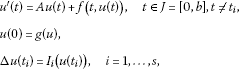(1.1)

where $A:D\left(A\right)\subseteq X\to X$ is the infinitesimal generator of a strongly continuous semigroup $T\left(t\right)$, $t\ge 0$ in a Banach space X, $0={t}_{0}<{t}_{1}<{t}_{2}<\cdots <{t}_{s}<{t}_{s+1}=b$, $\mathrm{\Delta }u\left({t}_{i}\right)=u\left({t}_{i}^{+}\right)-u\left({t}_{i}^{-}\right)$, $u\left({t}_{i}^{-}\right)$, $u\left({t}_{i}^{+}\right)$ denote the left and right limit of u at ${t}_{i}$, respectively. f, g, ${I}_{i}$ are appropriate functions to be specified later.

Impulsive differential equations are recognized as excellent models to study the evolution processes that are subject to sudden changes in their states; see the monographs of Lakshmikantham et al., Benchohra et al.. In recent years impulsive differential equations in Banach spaces have been investigated by many authors; see  and references therein. Liu  discussed the existence and uniqueness of mild solutions for a semilinear impulsive Cauchy problem with Lipschitz impulsive functions. Non-Lipschitzian impulsive equations are considered by Nieto et al.. Cardinali and Rubbioni  proved the existence of mild solutions for the impulsive Cauchy problem controlled by a semilinear evolution differential inclusion. In , Abada et al. studied the existence of integral solutions for some nondensely defined impulsive semilinear functional differential inclusions.

On the other hand, the study of abstract nonlocal initial value problems was initiated by Byszewski, and the importance of the problem consists in the fact that it is more general and has better effect than the classical initial conditions $u\left(0\right)={u}_{0}$ alone. Therefore, it has been studied extensively under various conditions. Here we mention some results. Byszewski and Lakshmikantham , Byszewski  obtained the existence and uniqueness of mild solutions and classical solutions in the case that Lipschitz-type conditions are satisfied. In , Fu and Ezzinbi studied neutral functional-differential equations with nonlocal conditions. Aizicovici , Xue [17, 18] discussed the case when A generates a nonlinear contraction semigroup on X and obtained the existence of integral solutions for nonlinear differential equations. In particular, the measure of noncompactness has been used as an important tool to deal with some similar functional differential and integral equations; see .

From the viewpoint of theory and practice, it is natural for mathematics to combine impulsive conditions and nonlocal conditions. Recently, the nonlocal impulsive differential problem of type (1.1) has been discussed in the papers of Liang et al. and Fan et al.[24, 25], where a semigroup $T\left(t\right)$ is supposed to be compact, and g is Lipschitz continuous, compact, and strongly continuous, respectively. Very recently, Zhu et al. obtain the existence results when a nonlocal item g is Lipschitz continuous by using the Hausdorff measure of noncompactness and operator transformation. Compared with the results in , in this paper we do not require the compactness of the semigroup $T\left(t\right)$ and Lipschitz continuity of f. More important, by using the property of the measure of noncompactness in $PC\left(\left[0,b\right];X\right)$ given by us (see Lemma 2.7), the impulsive conditions and nonlocal conditions can be considered in a unified way under various conditions, including compactness conditions, Lipschitz conditions and mixed-type conditions. Hence, our results generalize and partially improve the results in [23, 25, 26].

This paper is organized as follows. In Section 2, we present some concepts and facts about the strongly continuous semigroup and the measure of noncompactness. In Section 3, we give four existence theorems of the problem (1.1) by using a condensing operator and the measure of noncompactness. At last, an example of an impulsive partial differential system is given in Section 4.

## 2 Preliminaries

Let $\left(X,\parallel \cdot \parallel \right)$ be a real Banach space. We denote by $C\left(\left[0,b\right];X\right)$ the space of X-valued continuous functions on $\left[0,b\right]$ with the norm $\parallel x\parallel =sup\left\{\parallel x\left(t\right)\parallel ,t\in \left[0,b\right]\right\}$ and by ${L}^{1}\left(\left[0,b\right];X\right)$ the space of X-valued Bochner integrable functions on $\left[0,b\right]$ with the norm ${\parallel f\parallel }_{{L}^{1}}={\int }_{0}^{b}\parallel f\left(t\right)\parallel \phantom{\rule{0.2em}{0ex}}\mathrm{d}t$.

The semigroup $T\left(t\right)$ is said to be equicontinuous if $\left\{T\left(t\right)x:x\in B\right\}$ is equicontinuous at $t>0$ for any bounded subset $B\subset X$ (cf.). Obviously, if $T\left(t\right)$ is a compact semigroup, it must be equicontinuous. And the converse of the relation usually is not correct. Throughout this paper, we suppose that

(HA) The semigroup $\left\{T\left(t\right):t\ge 0\right\}$ generated by A is equicontinuous. Moreover, there exists a positive number M such that $M={sup}_{0\le t\le b}\parallel T\left(t\right)\parallel$.

For the sake of simplicity, we put $J=\left[0,b\right]$; ${J}_{0}=\left[0,{t}_{1}\right]$; ${J}_{i}=\left({t}_{i},{t}_{i+1}\right]$, $i=1,\dots ,s$. In order to define a mild solution of the problem (1.1), we introduce the set . It is easy to verify that $PC\left(\left[0,b\right];X\right)$ is a Banach space with the norm ${\parallel u\parallel }_{PC}=sup\left\{\parallel u\left(t\right)\parallel ,t\in \left[0,b\right]\right\}$.

Definition 2.1 A function $u\in PC\left(\left[0,b\right];X\right)$ is a mild solution of the problem (1.1) if

$u\left(t\right)=T\left(t\right)g\left(u\right)+{\int }_{0}^{t}T\left(t-s\right)f\left(s,u\left(s\right)\right)\phantom{\rule{0.2em}{0ex}}\mathrm{d}s+\sum _{0<{t}_{i}

for all $t\in \left[0,b\right]$.

Now, we introduce the Hausdorff measure of noncompactness (in short MNC) $\beta \left(\cdot \right)$ defined by

for each bounded subset B in a Banach space X. We recall the following properties of the Hausdorff measure of noncompactness β.

Lemma 2.2 ()

Let X be a real Banach space and$B,C\subseteq X$be bounded. Then the following properties are satisfied:

1. (1)

B is relatively compact if and only if $\beta \left(B\right)=0$;

2. (2)

$\beta \left(B\right)=\beta \left(\overline{B}\right)=\beta \left(convB\right)$, where $\overline{B}$ and convB mean the closure and convex hull of B, respectively;

3. (3)

$\beta \left(B\right)\le \beta \left(C\right)$ when $B\subseteq C$;

4. (4)

$\beta \left(B+C\right)\le \beta \left(B\right)+\beta \left(C\right)$, where $B+C=\left\{x+y:x\in B,y\in C\right\}$;

5. (5)

$\beta \left(B\cup C\right)\le max\left\{\beta \left(B\right),\beta \left(C\right)\right\}$;

6. (6)

$\beta \left(\lambda B\right)\le |\lambda |\beta \left(B\right)$ for any $\lambda \in R$;

7. (7)

If the map $Q:D\left(Q\right)\subseteq X\to Z$ is Lipschitz continuous with a constant k, then ${\beta }_{Z}\left(QB\right)\le k\beta \left(B\right)$ for any bounded subset $B\subseteq D\left(Q\right)$, where Z is a Banach space.

The map $Q:D\subseteq X\to X$ is said to be β-condensing if Q is continuous and bounded, and for any nonprecompact bounded subset $B\subset D$, we have $\beta \left(QB\right)<\beta \left(B\right)$, where X is a Banach space.

If$D\subset X$is bounded, closed, and convex, the continuous map$Q:D\to D$is β-condensing, then Q has at least one fixed point in D.

In order to remove the strong restriction on the coefficient in Darbo-Sadovskii’s fixed point theorem, Sun and Zhang  generalized the definition of a β-condensing operator. At first, we give some notation. Let $D\subset X$ be closed and convex, the map $Q:D\to D$ and ${x}_{0}\in D$. For every $B\subset D$, set

${Q}^{\left(1,{x}_{0}\right)}\left(B\right)=Q\left(B\right),\phantom{\rule{2em}{0ex}}{Q}^{\left(n,{x}_{0}\right)}\left(B\right)=Q\left(\overline{conv}\left\{{Q}^{\left(n-1,{x}_{0}\right)}B,{x}_{0}\right\}\right),$

where $\overline{conv}$ means the closure of convex hull, $n=2,3,\dots$ .

Definition 2.4 Let $D\subset X$ be closed and convex. The map $Q:D\to D$ is said to be β-convex-power condensing if Q is continuous, bounded and there exist ${x}_{0}\in D$, ${n}_{0}\in N$ such that for every nonprecompact bounded subset $B\subset D$, we have

$\beta \left({Q}^{\left({n}_{0},{x}_{0}\right)}\left(B\right)\right)<\beta \left(B\right).$

Obviously, if ${n}_{0}=1$, then a β-convex-power condensing operator is β-condensing. Thus, the convex-power condensing operator is a generalization of the condensing operator. Now, we give the fixed point theorem about the convex-power condensing operator.

Lemma 2.5 ()

If$D\subset X$is bounded, closed, and convex, the continuous map$Q:D\to D$is β-convex-power condensing, then Q has at least one fixed point in D.

Now, we give an important property of the Hausdorff MNC in $PC\left(\left[0,b\right];X\right)$, which is an extension to the property of MNC in $C\left(\left[0,b\right];X\right)$ and makes us deal with the impulsive differential equations from a unified perspective.

Lemma 2.6 ()

If$W\subseteq C\left(\left[0,b\right];X\right)$is bounded, then$\beta \left(W\left(t\right)\right)\le \beta \left(W\right)$for all$t\in \left[0,b\right]$, where$W\left(t\right)=\left\{u\left(t\right);u\in W\right\}\subseteq X$. Furthermore, if W is equicontinuous on$\left[0,b\right]$, then$\beta \left(W\left(t\right)\right)$is continuous on$\left[0,b\right]$and$\beta \left(W\right)=sup\left\{\beta \left(W\left(t\right)\right),t\in \left[0,b\right]\right\}$.

By applying Lemma 2.6, we shall extend the result to the space $PC\left(\left[0,b\right];X\right)$.

Lemma 2.7 If$W\subseteq PC\left(\left[0,b\right];X\right)$is bounded, then$\beta \left(W\left(t\right)\right)\le \beta \left(W\right)$for all$t\in \left[0,b\right]$, where$W\left(t\right)=\left\{u\left(t\right);u\in W\right\}\subseteq X$. Furthermore, suppose the following conditions are satisfied:

1. (1)

W is equicontinuous on ${J}_{0}=\left[0,{t}_{1}\right]$ and each ${J}_{i}=\left({t}_{i},{t}_{i+1}\right]$, $i=1,\dots ,s$;

2. (2)

W is equicontinuous at $t={t}_{i}^{+}$, $i=1,\dots ,s$.

Then${sup}_{t\in \left[0,b\right]}\beta \left(W\left(t\right)\right)=\beta \left(W\right)$.

Proof For arbitrary $\epsilon >0$, there exists ${W}_{i}\subseteq PC\left(\left[0,b\right];X\right)$, $1\le i\le n$, such that $W={\bigcup }_{i=1}^{n}{W}_{i}$ and

$diam\left({W}_{i}\right)\le 2\beta \left(W\right)+2\epsilon ,\phantom{\rule{1em}{0ex}}i=1,2,\dots ,n,$

where $diam\left(\cdot \right)$ denotes the diameter of a bounded set. Now, we have $W\left(t\right)={\bigcup }_{i=1}^{n}{W}_{i}\left(t\right)$ for each $t\in \left[a,b\right]$, and

$\parallel x\left(t\right)-y\left(t\right)\parallel \le \parallel x-y\parallel \le diam\left({W}_{i}\right)$

for $x,y\in {W}_{i}$. From the above two inequalities, it follows that

$2\beta \left(W\left(t\right)\right)\le diam\left({W}_{i}\left(t\right)\right)\le diam\left({W}_{i}\right)\le 2\beta \left(W\right)+2\epsilon .$

By the arbitrariness of ε, we get that $\beta \left(W\left(t\right)\right)\le \beta \left(W\right)$ for every $t\in \left[0,b\right]$. Therefore, we have ${sup}_{t\in \left[0,b\right]}\beta \left(W\left(t\right)\right)\le \beta \left(W\right)$.

Next, if the conditions (1) and (2) are satisfied, it remains to prove that $\beta \left(W\right)\le {sup}_{t\in \left[0,b\right]}\beta \left(W\left(t\right)\right)$. We denote $W{|}_{\overline{{J}_{i}}}$ by the restriction of W on $\overline{{J}_{i}}=\left[{t}_{i},{t}_{i+1}\right]$, $i=0,1,\dots ,s$. That is, for $x\in W{|}_{\overline{{J}_{i}}}$, define that

$x\left(t\right)=\left\{\begin{array}{cc}x\left(t\right),\hfill & {t}_{i}

and obviously $W{|}_{\overline{{J}_{i}}}$ is equicontinuous on $\overline{{J}_{i}}$ due to the conditions (1) and (2). Then from Lemma 2.6, we have that

$\beta \left(W{|}_{\overline{{J}_{i}}}\right)=\underset{t\in \overline{{J}_{i}}}{sup}\beta \left(W{|}_{\overline{{J}_{i}}}\left(t\right)\right).$

Moreover, we define the map

$\mathrm{\Lambda }:PC\left(\left[0,b\right];X\right)⟶C\left(\left[0,{t}_{1}\right];X\right)×C\left(\left[{t}_{1},{t}_{2}\right];X\right)×\cdots ×C\left(\left[{t}_{s},b\right];X\right)$

by $x\to \left({x}_{0},{x}_{1},\dots ,{x}_{s}\right)$, where $x\in PC\left(\left[0,b\right];X\right)$, ${x}_{i}=x{|}_{\overline{{J}_{i}}}$, $\parallel \left({x}_{0},{x}_{1},\dots ,{x}_{s}\right)\parallel ={max}_{0\le i\le s}\parallel {x}_{i}\parallel$. As Λ is an isometric mapping, noticing the equicontinuity of $W{|}_{\overline{{J}_{i}}}$ on $\overline{{J}_{i}}$, we have that

$\beta \left(W\right)=\beta \left(W{|}_{\overline{{J}_{0}}}×W{|}_{\overline{{J}_{1}}}×\cdots ×W{|}_{\overline{{J}_{s}}}\right)\le \underset{i}{max}\beta \left(W{|}_{\overline{{J}_{i}}}\right)=\underset{i}{max}\underset{t\in \overline{{J}_{i}}}{sup}\beta \left(W{|}_{\overline{{J}_{i}}}\left(t\right)\right).$

And from the fact that ${sup}_{t\in \overline{{J}_{i}}}\beta \left(W{|}_{\overline{{J}_{i}}}\left(t\right)\right)\le {sup}_{t\in \left[0,b\right]}\beta \left(W\left(t\right)\right)$, for each $i=0,\dots ,s$, we get that $\beta \left(W\right)\le {sup}_{t\in \left[0,b\right]}\beta \left(W\left(t\right)\right)$. This completes the proof. □

Lemma 2.8 ()

If$W\subset C\left(\left[0,b\right];X\right)$is bounded and equicontinuous, then$\beta \left(W\left(t\right)\right)$is continuous and

$\beta \left({\int }_{0}^{t}W\left(s\right)\phantom{\rule{0.2em}{0ex}}\mathrm{d}s\right)\le {\int }_{0}^{t}\beta \left(W\left(s\right)\right)\phantom{\rule{0.2em}{0ex}}\mathrm{d}s,$

for all$t\in \left[0,b\right]$, where${\int }_{0}^{t}W\left(s\right)\phantom{\rule{0.2em}{0ex}}\mathrm{d}s=\left\{{\int }_{0}^{t}x\left(s\right)\phantom{\rule{0.2em}{0ex}}\mathrm{d}s:x\in W\right\}$.

Lemma 2.9 If the hypothesis (HA) is satisfied, i.e., $\left\{T\left(t\right):t\ge 0\right\}$is equicontinuous, and$\eta \in {L}^{1}\left(\left[0,b\right];{R}^{+}\right)$, then the set$\left\{{\int }_{0}^{t}T\left(t-s\right)u\left(s\right)\phantom{\rule{0.2em}{0ex}}\mathrm{d}s:\parallel u\left(s\right)\parallel \le \eta \left(s\right)\phantom{\rule{0.5em}{0ex}}\mathit{\text{for a.e.}}\phantom{\rule{0.5em}{0ex}}s\in \left[0,b\right]\right\}$is equicontinuous for$t\in \left[0,b\right]$.

Proof We let $0\le t and have that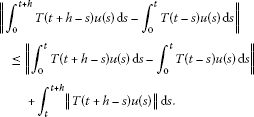(2.1)

If $t=0$, then the right-hand side of (2.1) can be made small when h is small independent of u. If $t>0$, then we can find a small $\epsilon >0$ with $t-\epsilon >0$. Then it follows from (2.1) that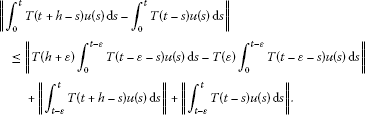(2.2)

Here, as $T\left(t\right)$ is equicontinuous for $t>0$, thus

uniformly for u.

Then from (2.1), (2.2) and the absolute continuity of integrals, we get that is equicontinuous for $t\in \left[0,b\right]$. □

## 3 Main results

In this section we give the existence results for the problem (1.1) under different conditions on g and ${I}_{i}$ when the semigroup is not compact and f is not compact or Lipschitz continuous, by using Lemma 2.7 and the generalized β-condensing operator. More precisely, Theorem 3.1 is concerned with the case that compactness conditions are satisfied. Theorem 3.4 deals with the case that Lipschitz conditions are satisfied. And mixed-type conditions are considered in Theorem 3.5 and Theorem 3.6.

Let r be a finite positive constant, and set ${B}_{r}=\left\{x\in X:\parallel x\parallel \le r\right\}$, ${W}_{r}=\left\{u\in PC\left(\left[0,b\right];X\right):u\left(t\right)\in {B}_{r},t\in \left[0,b\right]\right\}$. We define the solution map $G:PC\left(\left[0,b\right];X\right)\to PC\left(\left[0,b\right];X\right)$ by

$\left(Gu\right)\left(t\right)=T\left(t\right)g\left(u\right)+{\int }_{0}^{t}T\left(t-s\right)f\left(s,u\left(s\right)\right)\phantom{\rule{0.2em}{0ex}}\mathrm{d}s+\sum _{0<{t}_{i}
(3.1)

with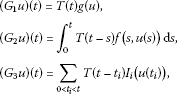for all $t\in \left[0,b\right]$. It is easy to see that u is the mild solution of the problem (1.1) if and only if u is a fixed point of the map G.

We list the following hypotheses:

(Hf) $f:\left[0,b\right]×X\to X$ satisfies the following conditions:

1. (i)

$f\left(t,\cdot \right):X\to X$ is continuous for a.e. $t\in \left[0,b\right]$ and $f\left(\cdot ,x\right):\left[0,b\right]\to X$ is measurable for all $x\in X$. Moreover, for any $r>0$, there exists a function ${\rho }_{r}\in {L}^{1}\left(\left[0,b\right],R\right)$ such that

$\parallel f\left(t,x\right)\parallel \le {\rho }_{r}\left(t\right)$

for a.e. $t\in \left[0,b\right]$ and $x\in {B}_{r}$.

1. (ii)

there exists a constant $L>0$ such that for any bounded set $D\subset X$,

$\beta \left(f\left(t,D\right)\right)\le L\beta \left(D\right)$
(3.2)

for a.e. $t\in \left[0,b\right]$.

(Hg1) $g:PC\left(\left[0,b\right];X\right)\to X$ is continuous and compact.

(HI1) ${I}_{i}:X\to X$ is continuous and compact for $i=1,\dots ,s$.

Theorem 3.1 Assume that the hypotheses (HA), (Hf), (Hg1), (HI1) are satisfied, then the nonlocal impulsive problem (1.1) has at least one mild solution on$\left[0,b\right]$provided that there exists a constant$r>0$such that

$M\left[\underset{u\in {W}_{r}}{sup}\parallel g\left(u\right)\parallel +{\parallel {\rho }_{r}\parallel }_{{L}^{1}}+\underset{u\in {W}_{r}}{sup}\sum _{i=1}^{s}\parallel {I}_{i}\left(u\left({t}_{i}\right)\right)\parallel \right]\le r.$
(3.3)

Proof We will prove that the solution map G has a fixed point by using the fixed point theorem about the β-convex-power condensing operator.

Firstly, we prove that the map G is continuous on $PC\left(\left[0,b\right];X\right)$. For this purpose, let ${\left\{{u}_{n}\right\}}_{n=1}^{\mathrm{\infty }}$ be a sequence in $PC\left(\left[0,b\right];X\right)$ with ${lim}_{n\to \mathrm{\infty }}{u}_{n}=u$ in $PC\left(\left[0,b\right];X\right)$. By the continuity of f with respect to the second argument, we deduce that for each $s\in \left[0,b\right]$, $f\left(s,{u}_{n}\left(s\right)\right)$ converges to $f\left(s,u\left(s\right)\right)$ in X. And we have

$\begin{array}{rcl}{\parallel G{u}_{n}-Gu\parallel }_{PC}& \le & M\left[\parallel g\left({u}_{n}\right)-g\left(u\right)\parallel +\sum _{i=1}^{s}\parallel {I}_{i}\left({u}_{n}\left({t}_{i}\right)\right)-{I}_{i}\left(u\left({t}_{i}\right)\right)\parallel \right]\\ +M{\int }_{0}^{b}\parallel f\left(s,{u}_{n}\left(s\right)\right)-f\left(s,u\left(s\right)\right)\parallel \phantom{\rule{0.2em}{0ex}}\mathrm{d}s.\end{array}$

Then by the continuity of g, ${I}_{i}$ and using the dominated convergence theorem, we get ${lim}_{n\to \mathrm{\infty }}G{u}_{n}=Gu$ in $PC\left(\left[0,b\right];X\right)$.

Secondly, we claim that $G{W}_{r}\subseteq {W}_{r}$. In fact, for any $u\in {W}_{r}\subset PC\left(\left[0,b\right];X\right)$, from (3.1) and (3.3), we have

$\begin{array}{rcl}\parallel \left(Gu\right)\left(t\right)\parallel & \le & \parallel T\left(t\right)g\left(u\right)\parallel +\parallel {\int }_{0}^{t}T\left(t-s\right)f\left(s,u\left(s\right)\right)\phantom{\rule{0.2em}{0ex}}\mathrm{d}s\parallel +\sum _{0<{t}_{i}

for each $t\in \left[0,b\right]$. It implies that $G{W}_{r}\subseteq {W}_{r}$.

Now, we show that $G{W}_{r}$ is equicontinuous on ${J}_{0}=\left[0,{t}_{1}\right]$, ${J}_{i}=\left({t}_{i},{t}_{i+1}\right]$ and is also equicontinuous at $t={t}_{i}^{+}$, $i=1,\dots ,s$. Indeed, we only need to prove that $G{W}_{r}$ is equicontinuous on $\left[{t}_{1},{t}_{2}\right]$, as the cases for other subintervals are the same. For $u\in {W}_{r}$, ${t}_{1}\le s, we have, using the semigroup property,

$\begin{array}{rcl}\parallel T\left(t\right)g\left(u\right)-T\left(s\right)g\left(u\right)\parallel & =& \parallel T\left(s\right)\left[T\left(t-s\right)-T\left(0\right)\right]g\left(u\right)\parallel \\ \le & M\parallel \left[T\left(t-s\right)-T\left(0\right)\right]g\left(u\right)\parallel .\end{array}$

Thus, ${G}_{1}{W}_{r}$ is equicontinuous on $\left[{t}_{1},{t}_{2}\right]$ due to the compactness of g and the strong continuity of $T\left(\cdot \right)$. The same idea can be used to prove the equicontinuity of ${G}_{3}{W}_{r}$ on $\left[{t}_{1},{t}_{2}\right]$. That is, for $u\in {W}_{r}$, ${t}_{1}\le s, we have

$\parallel T\left(t-{t}_{1}\right){I}_{1}\left(u\left({t}_{1}\right)\right)-T\left(s-{t}_{1}\right){I}_{1}\left(u\left({t}_{1}\right)\right)\parallel \le M\parallel \left[T\left(t-s\right)-T\left(0\right)\right]{I}_{1}\left(u\left({t}_{1}\right)\right)\parallel ,$

which implies the equicontinuity of ${G}_{3}{W}_{r}$ on $\left[{t}_{1},{t}_{2}\right]$ due to the compactness of ${I}_{1}$ and the strong continuity of $T\left(\cdot \right)$. Moreover, from Lemma 2.9, we have that ${G}_{2}{W}_{r}$ is equicontinuous on $\left[0,b\right]$. Therefore, we have that the functions in $G{W}_{r}=\left({G}_{1}+{G}_{2}+{G}_{3}\right){W}_{r}$ are equicontinuous on each $\left[{t}_{i},{t}_{i+1}\right]$, $i=0,1,\dots ,s$.

Set $W=\overline{conv}G\left({W}_{r}\right)$, where $\overline{conv}$ means the closure of convex hull. It is easy to verify that G maps W into itself and W is equicontiuous on each $\overline{{J}_{i}}=\left[{t}_{i},{t}_{i+1}\right]$, $i=0,1,\dots ,s$. Now, we show that $G:W\to W$ is a convex-power condensing operator. Take ${x}_{0}\in W$, we shall prove that there exists a positive integral ${n}_{0}$ such that

$\beta \left({G}^{\left({n}_{0},{x}_{0}\right)}\left(B\right)\right)<\beta \left(B\right),$

for every nonprecompact bounded subset $B\subset W$.

From Lemma 2.2 and Lemma 2.8, noticing the compactness of g and ${I}_{i}$, we have

$\begin{array}{rcl}\beta \left(\left({G}^{\left(1,{x}_{0}\right)}B\right)\left(t\right)\right)& =& \beta \left(\left(GB\right)\left(t\right)\right)\\ \le & \beta \left(T\left(t\right)g\left(B\right)\right)+\beta \left({\int }_{0}^{t}T\left(t-s\right)f\left(s,B\left(s\right)\right)\phantom{\rule{0.2em}{0ex}}\mathrm{d}s\right)\\ +\beta \left(\sum _{0<{t}_{i}

for $t\in \left[0,b\right]$. Further,

$\begin{array}{rcl}\beta \left(\left({G}^{\left(2,{x}_{0}\right)}B\right)\left(t\right)\right)& =& \beta \left(\left(G\overline{conv}\left\{{G}^{\left(1,{x}_{0}\right)}B,{x}_{0}\right\}\right)\left(t\right)\right)\\ \le & \beta \left(T\left(t\right)g\left(\overline{conv}\left\{{G}^{\left(1,{x}_{0}\right)}B,{x}_{0}\right\}\right)\right)\\ +\beta \left({\int }_{0}^{t}T\left(t-s\right)f\left(s,\overline{conv}\left\{{G}^{\left(1,{x}_{0}\right)}B\left(s\right),{x}_{0}\left(s\right)\right\}\right)\phantom{\rule{0.2em}{0ex}}\mathrm{d}s\right)\\ +\beta \left(\sum _{0<{t}_{i}

for $t\in \left[0,b\right]$. We can continue this iterative procedure and get that

$\beta \left(\left({G}^{\left(n,{x}_{0}\right)}B\right)\left(t\right)\right)\le \frac{{M}^{n}{L}^{n}{b}^{n}}{n!}\beta \left(B\right)$

for $t\in \left[0,b\right]$. As ${G}^{\left(n,{x}_{0}\right)}\left(B\right)$ is equicontinuous on each $\left[{t}_{i},{t}_{i+1}\right]$, by Lemma 2.7, we have that

$\beta \left({G}^{\left(n,{x}_{0}\right)}B\right)=\underset{t\in \left[0,b\right]}{sup}\beta \left(\left({G}^{\left(n,{x}_{0}\right)}B\right)\left(t\right)\right)\le \frac{{M}^{n}{L}^{n}{b}^{n}}{n!}\beta \left(B\right).$

By the fact that $\frac{{M}^{n}{L}^{n}{b}^{n}}{n!}\to 0$ as $n\to \mathrm{\infty }$, we know that there exists a large enough positive integral ${n}_{0}$ such that

$\frac{{M}^{{n}_{0}}{L}^{{n}_{0}}{b}^{{n}_{0}}}{{n}_{0}!}<1,$

which implies that $G:W\to W$ is a convex-power condensing operator. From Lemma 2.5, G has at least one fixed point in W, which is just a mild solution of the nonlocal impulsive problem (1.1). This completes the proof of Theorem 3.1. □

Remark 3.2 By using the method of the measure of noncompactness, we require f to satisfy some proper conditions of MNC, but do not require the compactness of a semigroup $T\left(t\right)$. Note that if f is compact or Lipschitz continuous, then the condition (Hf)(ii) is satisfied. And our work improves many previous results, where they need the compactness of $T\left(t\right)$ or f, or the Lipschitz continuity of f. In the proof, Lemma 2.7 plays an important role for the impulsive differential equations, which provides us with the way to calculate the measure of noncompactness in $PC\left(\left[0,b\right];X\right)$. The use of noncompact measures in functional differential and integral equations can also be seen in [1820, 22].

Remark 3.3 When we apply Darbo-Sadovskii’s fixed point theorem to get the fixed point of a map, a strong inequality is needed to guarantee its condensing property. By using the β-convex-power condensing operator developed by Sun et al., we do not impose any restrictions on the coefficient L. This generalized condensing operator also can be seen in Liu et al., where nonlinear Volterra integral equations are discussed.

In the following, by using Lemma 2.7 and Darbo-Sadovskii’s fixed point theorem, we give the existence results of the problem (1.1) under Lipschitz conditions and mixed-type conditions, respectively.

We give the following hypotheses:

(Hg2) $g:PC\left(\left[0,b\right];X\right)\to X$ is Lipschitz continuous with the Lipschitz constant k.

(HI2) ${I}_{i}:X\to X$ is Lipschitz continuous with the Lipschitz constant ${k}_{i}$; that is,

$\parallel {I}_{i}\left(x\right)-{I}_{i}\left(y\right)\parallel \le {k}_{i}\parallel x-y\parallel ,$

for $x,y\in X$, $i=1,\dots ,s$.

Theorem 3.4 Assume that the hypotheses (HA), (Hf), (Hg2), (HI2) are satisfied, then the nonlocal impulsive problem (1.1) has at least one mild solution on$\left[0,b\right]$provided that

$M\left(k+Lb+\sum _{i=1}^{s}{k}_{i}\right)<1$
(3.4)

and (3.3) are satisfied.

Proof From the proof of Theorem 3.1, we have that the solution operator G is continuous and maps ${W}_{r}$ into itself. It remains to show that G is β-condensing in ${W}_{r}$.

By the conditions (Hg2) and (HI2), we get that ${G}_{1}+{G}_{3}:{W}_{r}\to PC\left(\left[0,b\right];X\right)$ is Lipschitz continuous with the Lipschitz constant $M\left(k+{\sum }_{i=1}^{s}{k}_{i}\right)$. In fact, for $u,v\in {W}_{r}$, we have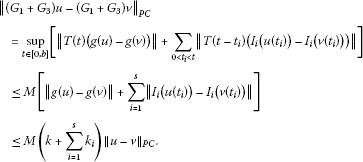Thus, from Lemma 2.2(7), we obtain that

$\beta \left(\left({G}_{1}+{G}_{3}\right){W}_{r}\right)\le M\left(k+\sum _{i=1}^{s}{k}_{i}\right)\beta \left({W}_{r}\right).$
(3.5)

For the operator $\left({G}_{2}u\right)\left(t\right)={\int }_{0}^{t}T\left(t-s\right)f\left(s,u\left(s\right)\right)\phantom{\rule{0.2em}{0ex}}\mathrm{d}s$, from Lemma 2.6, Lemma 2.8 and Lemma 2.9, we have

$\begin{array}{rcl}\beta \left({G}_{2}{W}_{r}\right)& =& \underset{t\in \left[0,b\right]}{sup}\beta \left(\left({G}_{2}{W}_{r}\right)\left(t\right)\right)\\ \le & \underset{t\in \left[0,b\right]}{sup}{\int }_{0}^{t}\beta \left(T\left(t-s\right)f\left(s,{W}_{r}\left(s\right)\right)\right)\phantom{\rule{0.2em}{0ex}}\mathrm{d}s\\ \le & \underset{t\in \left[0,b\right]}{sup}M{\int }_{0}^{t}L\beta \left({W}_{r}\left(s\right)\right)\phantom{\rule{0.2em}{0ex}}\mathrm{d}s\\ \le & MLb\beta \left({W}_{r}\right).\end{array}$
(3.6)

Combining (3.5) and (3.6), we have

$\begin{array}{rcl}\beta \left(G{W}_{r}\right)& \le & \beta \left(\left({G}_{1}+{G}_{3}\right){W}_{r}\right)+\beta \left({G}_{2}{W}_{r}\right)\\ \le & M\left(k+Lb+\sum _{i=1}^{s}{k}_{i}\right)\beta \left({W}_{r}\right).\end{array}$

From the condition (3.4), $M\left(k+Lb+{\sum }_{i=1}^{s}{k}_{i}\right)<1$, the solution map G is β-condensing in ${W}_{r}$. By Darbo-Sadovskii’s fixed point theorem, G has a fixed point in ${W}_{r}$, which is just a mild solution of the nonlocal impulsive problem (1.1). This completes the proof of Theorem 3.4. □

Among the previous works on nonlocal impulsive differential equations, few are concerned with the mixed-type conditions. Here, by using Lemma 2.7, we can also deal with the mixed-type conditions in a similar way.

Theorem 3.5 Assume that the hypotheses (HA), (Hf), (Hg1), (HI2) are satisfied, then the nonlocal impulsive problem (1.1) has at least one mild solution on$\left[0,b\right]$provided that

$M\left(Lb+\sum _{i=1}^{s}{k}_{i}\right)<1$
(3.7)

and (3.3) are satisfied.

Proof We will also use Darbo-Sadovskii’s fixed point theorem to obtain a fixed point of the solution operator G. From the proof of Theorem 3.1, we have that G is continuous and maps ${W}_{r}$ into itself.

Subsequently, we show that G is β-condensing in ${W}_{r}$. From the compactness of g and the strong continuity of $T\left(\cdot \right)$, we get that $\left\{T\left(\cdot \right)g\left(u\right):u\in {W}_{r}\right\}$ is equicontinuous on $\left[0,b\right]$. Then by Lemma 2.6, we have that

$\beta \left({G}_{1}{W}_{r}\right)=\underset{t\in \left[0,b\right]}{sup}\beta \left(\left({G}_{1}{W}_{r}\right)\left(t\right)\right)=\underset{t\in \left[0,b\right]}{sup}\beta \left(T\left(t\right)g\left({W}_{r}\right)\right)=0.$
(3.8)

On the other hand, for $u,v\in {W}_{r}$, we have

$\begin{array}{rcl}{\parallel {G}_{3}u-{G}_{3}v\parallel }_{PC}& =& \underset{t\in \left[0,b\right]}{sup}\parallel \sum _{0<{t}_{i}

Then by Lemma 2.2(7), we obtain that

$\beta \left({G}_{3}{W}_{r}\right)\le M\sum _{i=1}^{s}{k}_{i}\beta \left({W}_{r}\right).$
(3.9)

Combining (3.6), (3.8) and (3.9), we get that

$\beta \left(G{W}_{r}\right)\le \beta \left({G}_{1}{W}_{r}\right)+\beta \left({G}_{2}{W}_{r}\right)+\beta \left({G}_{3}{W}_{r}\right)\le M\left(Lb+\sum _{i=1}^{s}{k}_{i}\right)\beta \left({W}_{r}\right).$

From the condition (3.7), the map G is β-condensing in ${W}_{r}$. So, G has a fixed point in ${W}_{r}$ due to Darbo-Sadovskii’s fixed point theorem, which is just a mild solution of the nonlocal impulsive problem (1.1). This completes the proof of Theorem 3.5. □

Theorem 3.6 Assume that the hypotheses (HA), (Hf), (Hg2), (HI1) are satisfied, then the nonlocal impulsive problem (1.1) has at least one mild solution on$\left[0,b\right]$provided that

$M\left(k+Lb\right)<1$
(3.10)

and (3.3) are satisfied.

Proof From the proof of Theorem 3.1, we have that the solution operator G is continuous and maps ${W}_{r}$ into itself. In the following, we shall show that G is β-condensing in ${W}_{r}$.

By the Lipschitz continuity of g, we have that for $u,v\in {W}_{r}$,

${\parallel {G}_{1}u-{G}_{1}v\parallel }_{PC}=\underset{t\in \left[0,b\right]}{sup}\parallel T\left(t\right)\left[g\left(u\right)-g\left(v\right)\right]\parallel \le Mk{\parallel u-v\parallel }_{PC},$

which implies that

$\beta \left({G}_{1}{W}_{r}\right)\le Mk\beta \left({W}_{r}\right).$
(3.11)

Similar to the discussion in Theorem 3.1, from the compactness of ${I}_{i}$ and the strong continuity $T\left(\cdot \right)$, we get that ${G}_{3}{W}_{r}$ is equicontinuous on each $\overline{{J}_{i}}=\left[{t}_{i},{t}_{i+1}\right]$, $i=0,1,\dots ,s$. Then by Lemma 2.7, we have that

$\beta \left({G}_{3}{W}_{r}\right)=\underset{t\in \left[0,b\right]}{sup}\beta \left(\left({G}_{3}{W}_{r}\right)\left(t\right)\right)\le \sum _{i=1}^{s}\beta \left(T\left(t-{t}_{i}\right){I}_{i}\left({W}_{r}\left({t}_{i}\right)\right)\right)=0.$
(3.12)

Combining (3.6), (3.11) and (3.12), we have that

$\beta \left(G{W}_{r}\right)\le \beta \left({G}_{1}{W}_{r}\right)+\beta \left({G}_{2}{W}_{r}\right)+\beta \left({G}_{3}{W}_{r}\right)\le M\left(k+Lb\right)\beta \left({W}_{r}\right).$

From condition (3.10), the map G is β-condensing in ${W}_{r}$. So, G has a fixed point in ${W}_{r}$ due to Darbo-Sadovskii’s fixed point theorem, which is just a mild solution of the nonlocal impulsive problem (1.1). This completes the proof of Theorem 3.6. □

Remark 3.7 With the assumption of compactness on the associated semigroup, the existence of mild solutions to functional differential equations has been discussed in [6, 2325]. By using the method of the measure of noncompactness, we deal with the four cases of impulsive differential equations in a unified way and get the existence results when the semigroup in not compact.

## 4 An example

In the application to partial differential equations, such as a class of parabolic equations, the semigroup corresponding to the differential equations is an analytic semigroup. We know that an analytic semigroup or a compact semigroup must be equicontinuous; see Pazy . So, our results can be applied to these problems. If the operator $A=\theta$, the corresponding semigroup $T\left(t\right)=I$ is equicontinuous on $\left[0,b\right]$.

We consider the following partial differential system (based on ) to illustrate our abstract results:

$\left\{\begin{array}{c}\frac{\partial }{\partial t}\omega \left(t,x\right)=\frac{{\partial }^{2}}{\partial {x}^{2}}\omega \left(t,x\right)+F\left(t,\omega \left(t,x\right)\right),\phantom{\rule{1em}{0ex}}0\le t\le b,0\le x\le \pi ,\hfill \\ \omega \left(t,0\right)=\omega \left(t,\pi \right)=0,\hfill \\ \omega \left({t}_{i}^{+},x\right)-\omega \left({t}_{i}^{-},x\right)={I}_{i}\left(\omega \left({t}_{i},x\right)\right),\phantom{\rule{1em}{0ex}}i=1,\dots ,s,\hfill \\ \omega \left(0,x\right)=g\left(\omega \left(t,x\right)\right).\hfill \end{array}$

Take $X={L}^{2}\left[0,\pi \right]$ and the operator $A:D\left(A\right)\subseteq X\to X$ defined by $Az={z}^{″}$, with

From Pazy , we know that A is the infinitesimal generator of an analytic semigroup $T\left(t\right)$, $t\ge 0$. This implies that A satisfies the condition (HA).

Let $0<{t}_{1}<{t}_{2}<\cdots <{t}_{s}, $0<{s}_{1}<{s}_{2}<\cdots <{s}_{q}, ${c}_{j}\in R$ ($j=0,1,\dots ,q$), $h\left(\cdot \right)\in {L}^{1}\left(\left[0,b\right];R\right)$, ${\alpha }_{i}>0$ and ${\rho }_{i}\in C\left(\left[0,\pi \right]×\left[0,\pi \right],R\right)$ for $i=1,\dots ,s$. Now, we define that

1. (1)

$f\left(t,u\left(\xi \right)\right)={c}_{0}sin\left(u\left(\xi \right)\right)$, $t\in \left[0,b\right]$, $u\in X$.

2. (2)

$g\left(\omega \left(t,\xi \right)\right)={\sum }_{j=1}^{q}{c}_{j}\omega \left({s}_{j},\xi \right)$, $\omega \in PC\left(\left[0,b\right];X\right)$.

3. (3)

$g\left(\omega \left(t,\xi \right)\right)={\int }_{0}^{b}h\left(s\right)lg\left(1+|\omega \left(s,\xi \right)|\right)\phantom{\rule{0.2em}{0ex}}\mathrm{d}s$, $\omega \in PC\left(\left[0,b\right];X\right)$.

4. (4)

${I}_{i}\left(u\left(\xi \right)\right)=\frac{1}{{\alpha }_{i}|u\left(\xi \right)|+{t}_{i}}$, $u\in X$, $1\le i\le s$.

5. (5)

${I}_{i}\left(u\left(\xi \right)\right)={\int }_{0}^{\pi }{\rho }_{i}\left(\xi ,y\right){cos}^{2}\left(u\left(y\right)\right)\phantom{\rule{0.2em}{0ex}}\mathrm{d}y$, $u\in X$, $1\le i\le s$.

Then we obtain that

Case 1. Under the conditions (1) + (3) + (5), the assumptions in Theorem 3.1 are satisfied for large $r>0$. Therefore, the corresponding system (1.1) has at least a mild solution.

Case 2. Under the conditions (1) + (2) + (4), the assumptions in Theorem 3.4 are satisfied for large $r>0$. Therefore, the corresponding system (1.1) has at least a mild solution.

Case 3. Under the conditions (1) + (3) + (4), the assumptions in Theorem 3.5 are satisfied for large $r>0$. Therefore, the corresponding system (1.1) has at least a mild solution.

Case 4. Under the conditions (1) + (2) + (5), the assumptions in Theorem 3.6 are satisfied for large $r>0$. Therefore, the corresponding system (1.1) has at least a mild solution.

## References

1. Lakshmikantham V, Bainov DD, Simeonov PS: Theory of Impulsive Differential Equations. World Scientific, Singapore; 1989.

2. Benchohra M, Henderson J, Ntouyas SK: Impulsive Differential Equations and Inclusions. Hindawi Publishing, New York; 2006.

3. Guo M, Xue X, Li R: Controllability of impulsive evolution inclusions with nonlocal conditions. J. Optim. Theory Appl. 2004, 120: 355–374.

4. Hernández E, Rabelo M, Henríquez HR: Existence of solutions for impulsive partial neutral functional differential equations. J. Math. Anal. Appl. 2007, 331: 1135–1158. 10.1016/j.jmaa.2006.09.043

5. Ji S, Wen S: Nonlocal Cauchy problem for impulsive differential equations in Banach spaces. Int. J. Nonlinear Sci. 2010, 10(1):88–95.

6. Ji S, Li G: Existence results for impulsive differential inclusions with nonlocal conditions. Comput. Math. Appl. 2011, 62: 1908–1915. 10.1016/j.camwa.2011.06.034

7. Li J, Nieto JJ, Shen J: Impulsive periodic boundary value problems of first-order differential equations. J. Math. Anal. Appl. 2007, 325: 226–236. 10.1016/j.jmaa.2005.04.005

8. Benchohra M, Henderson J, Ntouyas SK: An existence result for first-order impulsive functional differential equations in Banach spaces. Comput. Math. Appl. 2001, 42: 1303–1310. 10.1016/S0898-1221(01)00241-3

9. Liu JH: Nonlinear impulsive evolution equations. Dyn. Contin. Discrete Impuls. Syst. 1999, 6: 77–85.

10. Nieto JJ, Rodriguez-Lopez R: Periodic boundary value problem for non-Lipschitzian impulsive functional differential equations. J. Math. Anal. Appl. 2006, 318: 593–610. 10.1016/j.jmaa.2005.06.014

11. Cardinali T, Rubbioni P: Impulsive semilinear differential inclusion: topological structure of the solution set and solutions on non-compact domains. Nonlinear Anal. 2008, 14: 73–84.

12. Abada N, Benchohra M, Hammouche H: Existence and controllability results for nondensely defined impulsive semilinear functional differential inclusions. J. Differ. Equ. 2009, 246: 3834–3863. 10.1016/j.jde.2009.03.004

13. Byszewski L, Lakshmikantham V: Theorem about the existence and uniqueness of solutions of a nonlocal Cauchy problem in a Banach space. Appl. Anal. 1990, 40: 11–19.

14. Byszewski L: Theorems about the existence and uniqueness of solutions of a semilinear evolution nonlocal Cauchy problem. J. Math. Anal. Appl. 1991, 162: 494–505. 10.1016/0022-247X(91)90164-U

15. Fu X, Ezzinbi K: Existence of solutions for neutral functional differential evolution equations with nonlocal conditions. Nonlinear Anal. 2003, 54: 215–227. 10.1016/S0362-546X(03)00047-6

16. Aizicovici S, McKibben M: Existence results for a class of abstract nonlocal Cauchy problems. Nonlinear Anal. 2000, 39: 649–668. 10.1016/S0362-546X(98)00227-2

17. Xue X: Nonlinear differential equations with nonlocal conditions in Banach spaces. Nonlinear Anal. 2005, 63: 575–586. 10.1016/j.na.2005.05.019

18. Xue X: Nonlocal nonlinear differential equations with a measure of noncompactness in Banach spaces. Nonlinear Anal. 2009, 70: 2593–2601. 10.1016/j.na.2008.03.046

19. Banas J, Zajac T: A new approach to the theory of functional integral equations of fractional order. J. Math. Anal. Appl. 2011, 375: 375–387. 10.1016/j.jmaa.2010.09.004

20. Cardinali T, Rubbioni P: On the existence of mild solutions of semilinear evolution differential inclusions. J. Math. Anal. Appl. 2005, 308: 620–635. 10.1016/j.jmaa.2004.11.049

21. Dong Q, Li G: Existence of solutions for semilinear differential equations with nonlocal conditions in Banach spaces. Electron. J. Qual. Theory Differ. Equ. 2009., 2009: Article ID 47

22. Agarwal RP, Benchohra M, Seba D: On the application of measure of noncompactness to the existence of solutions for fractional differential equations. Results Math. 2009, 55: 221–230. 10.1007/s00025-009-0434-5

23. Liang J, Liu JH, Xiao TJ: Nonlocal impulsive problems for nonlinear differential equations in Banach spaces. Math. Comput. Model. 2009, 49: 798–804. 10.1016/j.mcm.2008.05.046

24. Fan Z: Impulsive problems for semilinear differential equations with nonlocal conditions. Nonlinear Anal. 2010, 72: 1104–1109. 10.1016/j.na.2009.07.049

25. Fan Z, Li G: Existence results for semilinear differential equations with nonlocal and impulsive conditions. J. Funct. Anal. 2010, 258: 1709–1727. 10.1016/j.jfa.2009.10.023

26. Zhu L, Dong Q, Li G: Impulsive differential equations with nonlocal conditions in general Banach spaces. Adv. Differ. Equ. 2012., 2012: Article ID 10

27. Barbu V: Nonlinear Semigroups and Differential Equations in Banach Spaces. Noordhoff, Leyden; 1976.

28. Banas J, Goebel K Lect. Notes Pure Appl. Math. 60. In Measure of Noncompactness in Banach Spaces. Dekker, New York; 1980.

29. Sun J, Zhang X: The fixed point theorem of convex-power condensing operator and applications to abstract semilinear evolution equations. Acta Math. Sin. Chin. Ser. 2005, 48: 439–446.

30. Liu LS, Guo F, Wu CX, Wu YH: Existence theorems of global solutions for nonlinear Volterra type integral equations in Banach spaces. J. Math. Anal. Appl. 2005, 309: 638–649. 10.1016/j.jmaa.2004.10.069

31. Pazy A: Semigroups of Linear Operators and Applications to Partial Differential Equations. Springer, New York; 1983.

## Acknowledgements

Research is partially supported by the National Natural Science Foundation of China (11271316), the Postgraduate Innovation Project of Jiangsu Province (No. CXZZ12-0890), the NSF of China (11101353), the first author is also supported by the Youth Teachers Foundation of Huaiyin Institute of Technology (2012).

## Author information

Authors

### Corresponding author

Correspondence to Shaochun Ji.

### Competing interests

The authors declare that they have no competing interests.

### Authors’ contributions

All authors read and approved the final manuscript.

## Rights and permissions

Reprints and Permissions

Ji, S., Li, G. A unified approach to nonlocal impulsive differential equations with the measure of noncompactness. Adv Differ Equ 2012, 182 (2012). https://doi.org/10.1186/1687-1847-2012-182

• Accepted:

• Published:

• DOI: https://doi.org/10.1186/1687-1847-2012-182

### Keywords

• impulsive conditions
• nonlocal conditions
• Hausdorff measure of noncompactness
• fixed point theorem